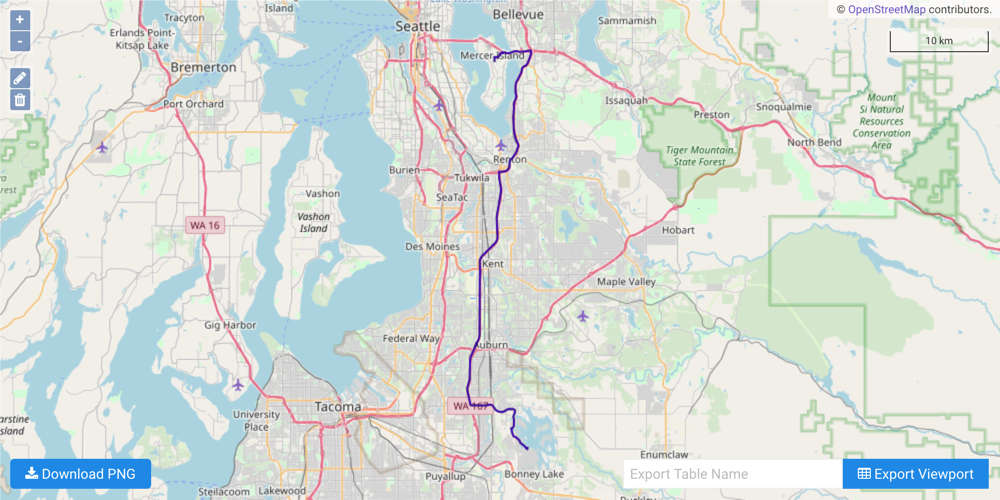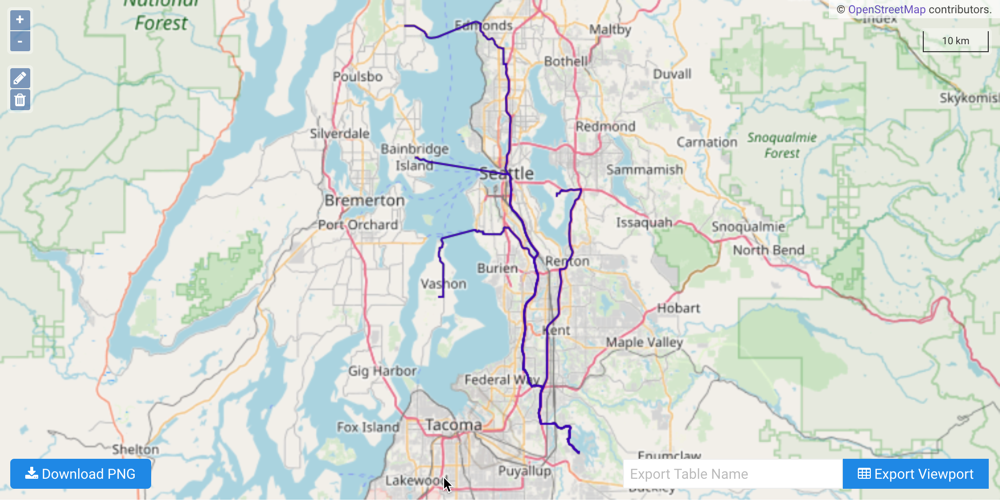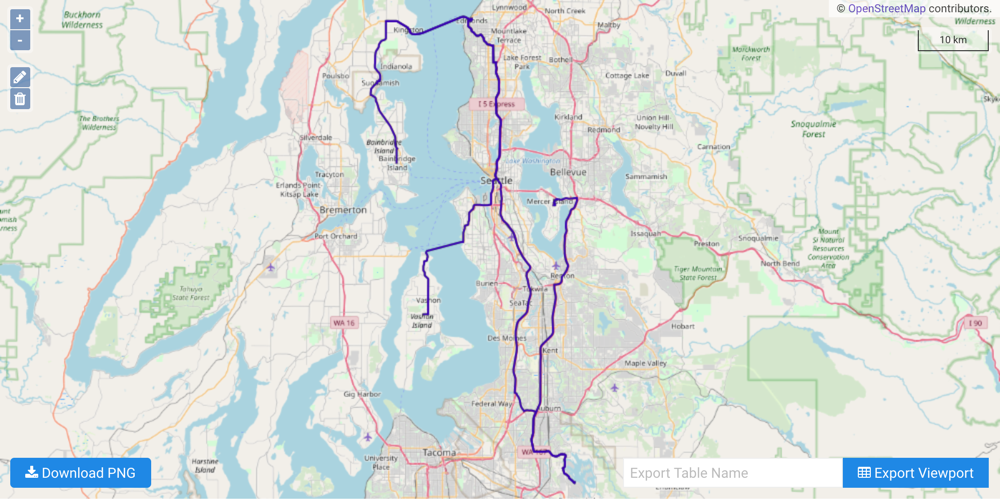# Shortest Path with Python

A Shortest Path graph solver example with the Python API

The following is a complete example, using the Python API, of solving a graph created with Seattle road network data for a shortest path problem via the /solve/graph endpoint. For more information on Network Graphs & Solvers, see Network Graphs & Solvers Concepts.

## Prerequisites

The prerequisites for running the shortest path solve graph example are listed below:

### Python API Installation

The native Kinetica Python API is accessible through the following means:

#### PyPI

The Python package manager, pip, is required to install the API from PyPI.

1. Install the API:

 ``````1 `````` ``````pip install gpudb --upgrade ``````
2. Test the installation:

 ``````1 `````` ``````python -c "import gpudb;print('Import Successful')" ``````

If Import Successful is displayed, the API has been installed as is ready for use.

#### Git

1. In the desired directory, run the following, but be sure to replace <kinetica-version> with the name of the installed Kinetica version, e.g., v7.1:

 ``````1 `````` ``````git clone -b release/ --single-branch https://github.com/kineticadb/kinetica-api-python.git ``````

 ``````1 `````` ``````cd kinetica-api-python ``````
3. In the root directory of the unzipped repository, install the Kinetica API:

 ``````1 `````` ``````sudo python setup.py install ``````
4. Test the installation (Python 2.7 (or greater) is necessary for running the API example):

 ``````1 `````` ``````python examples/example.py ``````

### Data File

The example script references the `road_weights.csv` data file, mentioned in the Prerequisites, in the current local directory, by default. This directory can specified as a parameter when running the example script.

## Script Detail

This example is going to demonstrate solving (both individual and batch solve) for the shortest path between source points and several destination points located within a road network in Seattle.

### Constants

Several constants are defined at the beginning of the script:

• SCHEMA -- the name of the schema in which the tables supporting the graph creation and match operations will be created

Important

The schema is created during the table setup portion of the script because the schema must exist prior to creating the tables that will later support the graph creation and match operations.

• TABLE_SRN -- the name of the table into which the Seattle road network dataset is loaded

• GRAPH_S -- the Seattle road network graph

• TABLE_GRAPH_S_SPSOLVED / TABLE_GRAPH_S_SPSOLVED2 / TABLE_GRAPH_S_SPSOLVED3 -- the Seattle road network graph shortest path solution tables

Constant Definitions
 ``````1 2 3 4 5 6 7 `````` ``````SCHEMA = "graph_s_seattle_shortest_path" TABLE_SRN = SCHEMA + ".seattle_road_network" GRAPH_S = SCHEMA + ".seattle_road_network_graph" TABLE_GRAPH_S_SPSOLVED = GRAPH_S + "_shortest_path_solved" TABLE_GRAPH_S_SPSOLVED2 = GRAPH_S + "_shortest_path_solved2" TABLE_GRAPH_S_SPSOLVED3 = GRAPH_S + "_shortest_path_solved3" ``````

### Graph Creation

One graph is used for the shortest path solve graph example utilized in the script: seattle_road_network_graph, a graph based on the road_weights dataset (the CSV file mentioned in Prerequisites).

The GRAPH_S graph is created with the following characteristics:

• It is directed because the roads in the graph have directionality (one-way and two-way roads)
• It has no explicitly defined nodes because the example relies on implicit nodes attached to the defined edges
• The edges are identified by WKTLINE, using WKT LINESTRINGs from the WKTLINE column of the seattle_road_network table. The road segments' directionality (DIRECTION) is derived from the TwoWay column of the seattle_road_network table.
• The weights (VALUESPECIFIED) are represented using the time taken to travel the segment found in the time column of the seattle_road_network table. The weights are matched to the edges using the same WKTLINE column as edges (EDGE_WKTLINE) and the same TwoWay column as the edge direction (EDGE_DIRECTION).
• It has no inherent restrictions for any of the nodes or edges in the graph
• It will be replaced with this instance of the graph if a graph of the same name exists (recreate)
 `````` 1 2 3 4 5 6 7 8 9 10 11 12 13 14 15 16 17 18 `````` ``````create_s_graph_response = kinetica.create_graph( graph_name = GRAPH_S, directed_graph = True, nodes = [], edges = [ TABLE_SRN + ".WKTLINE AS WKTLINE", TABLE_SRN + ".TwoWay AS DIRECTION" ], weights = [ TABLE_SRN + ".WKTLINE AS EDGE_WKTLINE", TABLE_SRN + ".TwoWay AS EDGE_DIRECTION", TABLE_SRN + ".time AS VALUESPECIFIED" ], restrictions = [], options = { "recreate": "true" } ) ``````

### Shortest Path

#### Single Source to Single Destination

The first example illustrates a simple shortest path solve from a single source node to a single destination node. First, the source node and destination node are defined.

Define Beginning & Ending Points
 ``````1 2 `````` ``````source_nodes = ["POINT(-122.1792501 47.2113606)"] destination_nodes = ["POINT(-122.2221 47.5707)"] ``````

Next, the seattle_road_network_graph graph is solved with the solve results being exported to the response:

Solve Graph for Shortest Path
 ``````1 2 3 4 5 6 7 `````` ``````kinetica.solve_graph( graph_name = GRAPH_S, solver_type = "SHORTEST_PATH", source_nodes = source_nodes, destination_nodes = destination_nodes, solution_table = TABLE_GRAPH_S_SPSOLVED ) ``````

The cost for the source node to visit the destination node is represented as time in minutes:

Solve Graph for Shortest Path Solution
 ``````1 2 3 4 5 `````` ``````+-------------------------------------------+---------------------+ | Destination Node | Cost (in minutes) | |-------------------------------------------+---------------------| | POINT (-122.22286015749 47.5709295272827) | 40.6023 | +-------------------------------------------+---------------------+ ``````

The solution output to WMS:#### Single Source to Many Destinations

The second example illustrates a shortest path solve from a single source node to many destination nodes. First, the source node and destination nodes are defined. When one source node and many destination nodes are provided, the graph solver will calculate a shortest path solve for each destination node.

Define Beginning & Multiple Ending Points
 ``````1 2 3 4 5 6 7 `````` ``````source_nodes = ["POINT(-122.1792501 47.2113606)"] destination_nodes = [ "POINT(-122.222100 47.570700)", "POINT(-122.541017 47.809121)", "POINT(-122.520440 47.624725)", "POINT(-122.467915 47.427280)" ] ``````

Next, the seattle_road_network_graph graph is solved with the solve results being exported to the response

Solve Graph for Shortest Paths with Multiple Destinations
 ``````1 2 3 4 5 6 7 `````` ``````kinetica.solve_graph( graph_name = GRAPH_S, solver_type = "SHORTEST_PATH", source_nodes = source_nodes, destination_nodes = destination_nodes, solution_table = TABLE_GRAPH_S_SPSOLVED2 ) ``````

The cost for the source node to visit each of the destination nodes separately is represented as time in minutes:

Solve Graph for Shortest Paths with Multiple Destinations Solution
 ``````1 2 3 4 5 6 7 8 `````` ``````+--------------------------------------------+---------------------+ | Destination Node | Cost (in minutes) | |--------------------------------------------+---------------------| | POINT (-122.22286015749 47.5709295272827) | 40.6023 | | POINT (-122.471138834953 47.4291801452637) | 69.5329 | | POINT (-122.519598305225 47.6248499751091) | 79.7307 | | POINT (-122.541549503803 47.8095200657845) | 90.872 | +--------------------------------------------+---------------------+ ``````

The solutions output to WMS:#### Many Sources to Many Destinations

The third example illustrates a shortest path solve from a many source nodes to many destination nodes. First, the source node and destination nodes are defined. If many source node and many destination nodes are provided, the graph solver will pair the source and destination node by list index and calculate a shortest path solve for each pair. For this example, there are two starting points (POINT(-122.1792501 47.2113606) and POINT(-122.375180125237 47.8122103214264)) and paths will be calculated from the first source to two different destinations and from the second source to two other destinations.

Important

Calculations from multiple unique sources are faster and more efficient than calculations with one unique source, but results may differ slightly between multiple unique source calculations and single unique source calculations (less than ~1% variance).

Define Multiple Beginning & Multiple Ending Points
 `````` 1 2 3 4 5 6 7 8 9 10 11 12 `````` ``````source_nodes = [ "POINT(-122.1792501 47.2113606)", "POINT(-122.1792501 47.2113606)", "POINT(-122.375180125237 47.8122103214264)", "POINT(-122.375180125237 47.8122103214264)" ] destination_nodes = [ "POINT(-122.222100 47.570700)", "POINT(-122.541017 47.809121)", "POINT(-122.520440 47.624725)", "POINT(-122.467915 47.427280)" ] ``````

Next, the seattle_road_network_graph graph is solved with the solve results being exported to the response:

Solve Graph for Shortest Paths with Multiple Sources & Multiple Destinations
 ``````1 2 3 4 5 6 7 `````` ``````kinetica.solve_graph( graph_name = GRAPH_S, solver_type = "SHORTEST_PATH", source_nodes = source_nodes, destination_nodes = destination_nodes, solution_table = TABLE_GRAPH_S_SPSOLVED3 ) ``````

The cost for the source node to visit each of the destination nodes separately is represented as time in minutes:

Solve Graph for Shortest Paths with Multiple Sources & Multiple Destinations Solution
 ``````1 2 3 4 5 6 7 8 `````` ``````+--------------------------------------------+--------------------------------------------+---------------------+ | Source Node | Destination Node | Cost (in minutes) | |--------------------------------------------+--------------------------------------------+---------------------| | POINT (-122.179250121117 47.2113606333733) | POINT (-122.221578061581 47.5709509849548) | 40.6791 | | POINT (-122.375180125237 47.8122103214264) | POINT (-122.520979642868 47.6248607039452) | 51.1339 | | POINT (-122.375180125237 47.8122103214264) | POINT (-122.471138834953 47.4291801452637) | 60.8013 | | POINT (-122.179250121117 47.2113606333733) | POINT (-122.541549503803 47.8095200657845) | 90.2997 | +--------------------------------------------+--------------------------------------------+---------------------+ ``````

The solutions output to WMS:• the `solve_graph_seattle_shortest_path.py` script is in the current directory
• the `road_weights.csv` file is in the current directory or use the data_dir parameter to specify the local directory containing it
 ``````1 `````` ``````python solve_graph_seattle_shortest_path.py [--url ] --username --password [--data_dir ] ``````